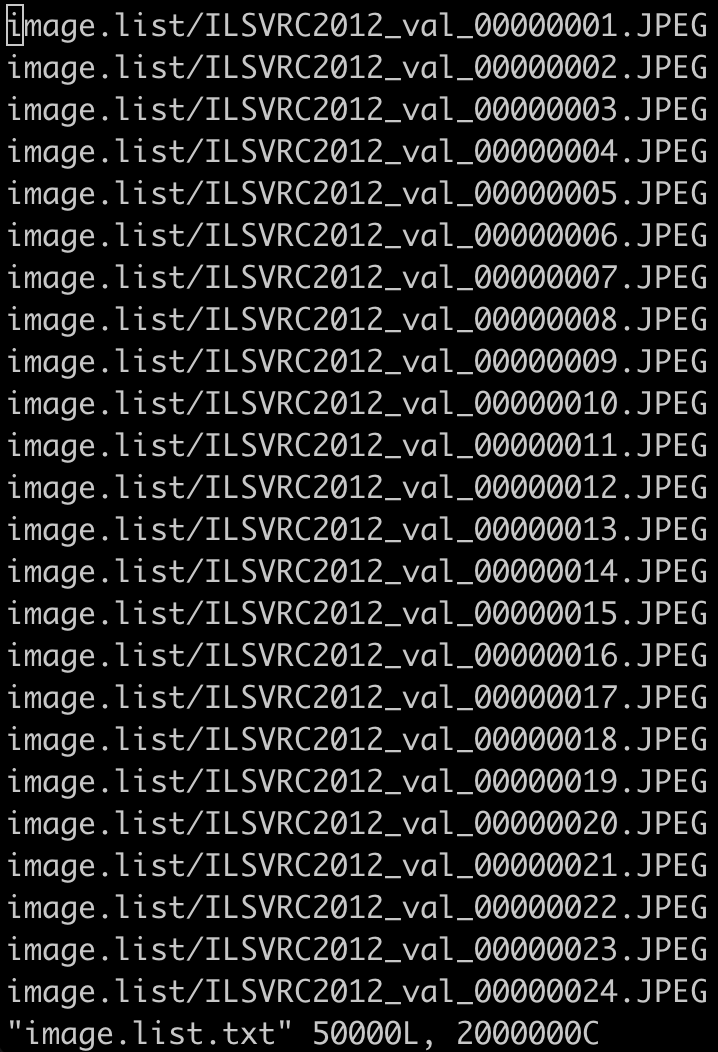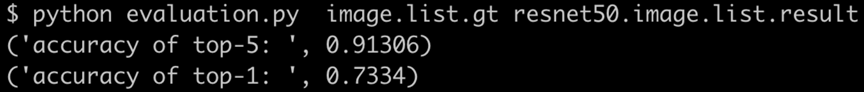# Model Accuracy Test - 3.5 English

## Vitis AI Library User Guide (UG1354)

Document ID
UG1354
Release Date
2023-06-29
Version
3.5 English

To test the model accuracy on the board, you must take into account the following factors.

• Image dataset
• Model
• Accuracy test program
• Ground truth file of the image dataset
• Accuracy extraction comparison script

## ResNet50 Example

Take resnet50 as an example.

1. Get the image dataset and the ground truth file of the dataset.

You can get the image dataset information from the Model Zoo content on GitHub. resnet50 uses the imagenet dataset.

2. Get the model.
``wget https://www.xilinx.com/bin/public/openDownload?filename=resnet_v1_50_tf-vek280-r3.5.0.tar.gz -O resnet_v1_50_tf-vek280-r3.5.0.tar.gz``
3. Copy the model to the board.
``scp resnet_v1_50_tf-vek280-r3.5.0.tar.gz root@IP_OF_BOARD:~/``

It includes resnet50 and resnet50_acc models.

4. Untar it on the board.
``tar -xzvf resnet_v1_50_tf-vek280-r3.5.0.tar.gz -C /usr/share/vitis-ai-library/models``
5. Set the path and image name of the image dataset to a file, such as image.list.txt.6. Run the accuracy test program on the board.
``````cd ~/Vitis-AI/examples/vai_library/samples/classification/
./test_accuracy_classification_mt resnet_v1_50_tf image.list.txt resnet50.image.list.result``````
Note: The accuracy test loads the resnet_v1_50_tf_acc model besides the resnet_v1_50_tf model. The only difference between the two models is the model prototxt file.

After the accuracy test program running finished, the result file resnet50.image.list.result will be generated.

7. Copy the resnet50.image.list.result to the host.
8. Run the corresponding accuracy extraction comparison script to get the final accuracy.
``python evaluation.py image.list.gt resnet50.image.list.result``The following is the code of evaluation.py

``````
#!/usr/bin/env python

import sys
# argv must groundtruth

dic_val={}

m = 0
temp = line.strip('/').split()
key = temp
value = int(temp)
dic_val[key] = value
m = m + 1

n = 0
temp = line1.strip('/').split()
if temp in dic_val and int(temp) == dic_val[temp]:
# print int(temp), dic_val[temp]
n = n + 1

#print m
#print n
rate = float(n)/float(m)
print("accuracy of top-5: ", rate)

l = 0
a = 0